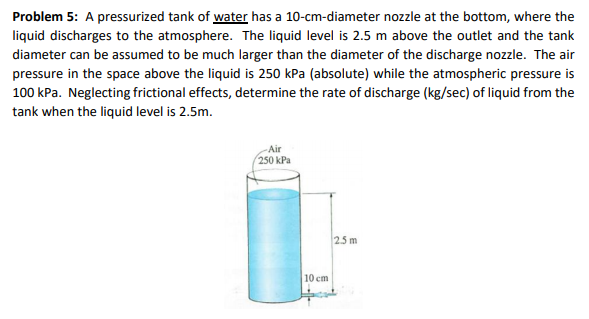# Problem 5: A pressurized tank of water has a 10-cm-diameter nozzle at the bottom, where the liquid discharges to the atmosphere. The liquid level is 2.5 m above the outlet and the tank diameter can be assumed to be much larger than the diameter of the discharge nozzle. The air pressure in the space above the liquid is 250 kPa (absolute) while the atmospheric pressure is 100 kPa. Neglecting frictional effects, determine the rate of discharge (kg/sec) of liquid from the tank when the liquid level is 2.5m. Air 250 kPa 25 m 10 cm

Question

How will you use the information supplied regarding,
a.The pressure in the air space at the top of the tank:
b.The atmospheric pressure:help_outlineImage TranscriptioncloseProblem 5: A pressurized tank of water has a 10-cm-diameter nozzle at the bottom, where the liquid discharges to the atmosphere. The liquid level is 2.5 m above the outlet and the tank diameter can be assumed to be much larger than the diameter of the discharge nozzle. The air pressure in the space above the liquid is 250 kPa (absolute) while the atmospheric pressure is 100 kPa. Neglecting frictional effects, determine the rate of discharge (kg/sec) of liquid from the tank when the liquid level is 2.5m. Air 250 kPa 25 m 10 cm fullscreen